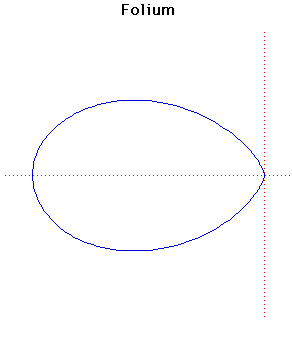# Curves

### FoliumCartesian equation:
$(x^{2} + y^{2})(y^{2} + x(x + b)) = 4axy^{2}$
Polar equation:
$r = -b \cos \theta + 4a \cos \theta \sin^{2} \theta$

### Description

The general form of the folium is given by the formula above. The word folium means leaf-shaped.

There are three special forms of the folium, the simple folium, the double folium and the trifolium. These correspond to the cases
$b = 4a, b = 0, b = a$
respectively in the formula above.

The graph plotted above is the simple folium. There are separate entries for the double folium and the trifolium.

The simple folium is the pedal curve of the tricuspoid where the pedal point is one of the cusps.

### Associated Curves

Definitions of the Associated curves